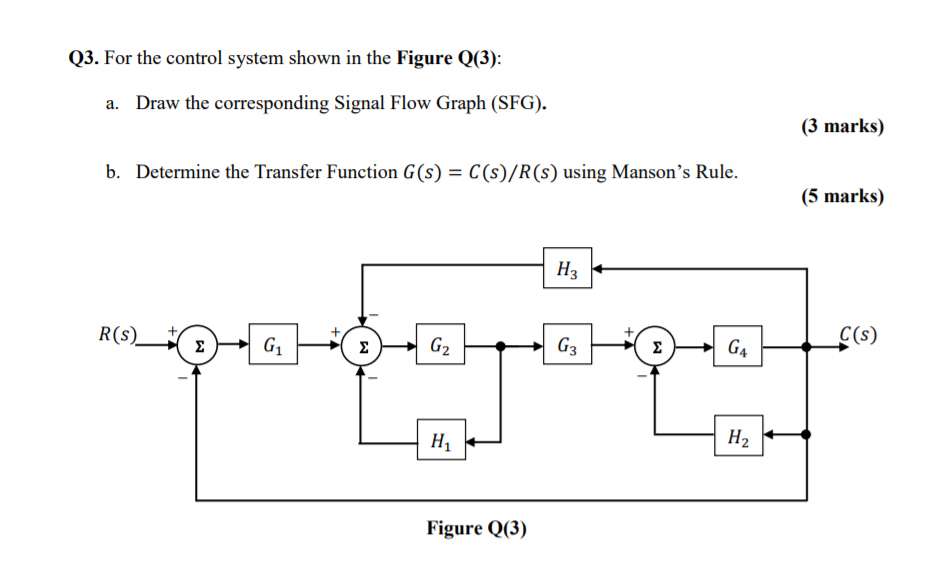# Question Q3. For the control system shown in the Figure Q(3): a. Draw the corresponding Signal Flow Graph (SFG). (3 marks) b. Determine the Transfer Function G(s) = C(s)/R(s) using Manson’s Rule. (5 marks) H R(S) C(s) Σ -G G2 G3 Σ G4 H H2 Figure Q(3)AO5IF6 The Asker · Electrical EngineeringTranscribed Image Text: Q3. For the control system shown in the Figure Q(3): a. Draw the corresponding Signal Flow Graph (SFG). (3 marks) b. Determine the Transfer Function G(s) = C(s)/R(s) using Manson’s Rule. (5 marks) H R(S) C(s) Σ -G G2 G3 Σ G4 H H2 Figure Q(3)
More
Transcribed Image Text: Q3. For the control system shown in the Figure Q(3): a. Draw the corresponding Signal Flow Graph (SFG). (3 marks) b. Determine the Transfer Function G(s) = C(s)/R(s) using Manson’s Rule. (5 marks) H R(S) C(s) Σ -G G2 G3 Σ G4 H H2 Figure Q(3)